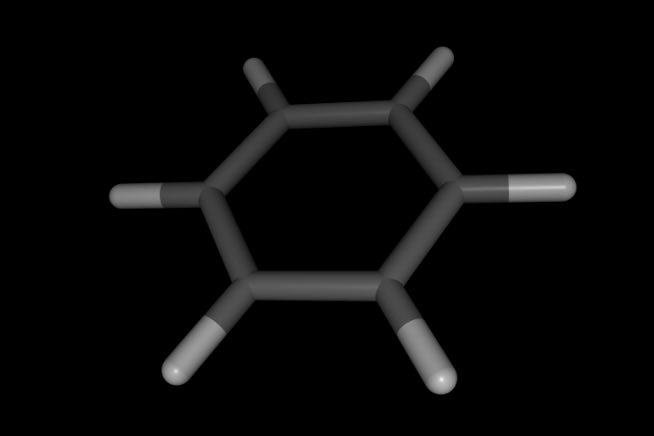# Creating Molecules from Smiles Strings#

Smiles strings provide a convenient way to represent a molecule as text.

You can create a molecule from a smiles string using the `sire.smiles()` function.

```>>> import sire as sr
>>> mol = sr.smiles("C1:C:C:C:C:C1")
>>> print(mol.atoms())
Selector<SireMol::Atom>( size=12
0:  Atom( C1:1    [  -0.92,   -1.05,    0.02] )
1:  Atom( C2:2    [  -1.37,    0.27,   -0.04] )
2:  Atom( C3:3    [  -0.45,    1.32,   -0.06] )
3:  Atom( C4:4    [   0.92,    1.05,   -0.02] )
4:  Atom( C5:5    [   1.37,   -0.27,    0.04] )
...
7:  Atom( H8:8    [  -2.43,    0.48,   -0.07] )
8:  Atom( H9:9    [  -0.80,    2.35,   -0.11] )
9:  Atom( H10:10  [   1.63,    1.87,   -0.04] )
10:  Atom( H11:11  [   2.43,   -0.48,    0.07] )
11:  Atom( H12:12  [   0.80,   -2.35,    0.11] )
)
>>> mol.view()
```Note how hydrogen atoms and coordinates of all atoms have been generated automatically. You can control this using the `add_hydrogens` and `generate_coordinates` options, e.g.

```>>> mol = sr.smiles("C1:C:C:C:C:C1", generate_coordinates=False)
>>> print(mol.atoms())
Selector<SireMol::Atom>( size=12
0:  Atom( C1:1 )
1:  Atom( C2:2 )
2:  Atom( C3:3 )
3:  Atom( C4:4 )
4:  Atom( C5:5 )
...
7:  Atom( H8:8 )
8:  Atom( H9:9 )
9:  Atom( H10:10 )
10:  Atom( H11:11 )
11:  Atom( H12:12 )
)
```
```>>> mol = sr.smiles("C1:C:C:C:C:C1", add_hydrogens=False)
>>> print(mol.atoms())
Selector<SireMol::Atom>( size=6
0:  Atom( C1:1 )
1:  Atom( C2:2 )
2:  Atom( C3:3 )
3:  Atom( C4:4 )
4:  Atom( C5:5 )
5:  Atom( C6:6 )
)
```

Note

Note that coordinates cannot be generated if `add_hydrogens` is `False`

The above code works by using the Chem.MolFromSmiles function from rdkit. This is used to create an rdkit Molecule which is converted to a `sire` `Molecule` using the functions in the `convert` module.

Note

Note that the default is that all sanitization steps provided by rdkit will be performed when generating the molecule. If any fail, then an exception will be raised and the molecule won’t be generated. You can change this behaviour by passing `must_sanitize` as `False`. This will run as many of the sanitization steps as possible, ignoring errors caused by individual steps.

## Generating smiles strings from molecules#

We can also convert in the other direction, e.g. from a `Molecule` to an rdkit Molecule. This can be used with rdkit’s MolToSmiles function to generate a smiles string. This is performed automatically using a molecule’s `.smiles()` function, e.g.

```>>> mol = sr.smiles("C1:C:C:C:C:C1")
>>> print(mol.smiles())
c1ccccc1
```

Note how hydrogens have been left out from the smiles string. They are only included if they are needed to resolve any ambiguity in the structure or chirality. For example;

```>>> mol = sr.smiles("C[C@H](N)C(=O)O")
>>> print(mol.smiles())
C[C@H](N)C(=O)O
```

You can ask for all of the hydrogens to be included explicitly by passing `include_hydrogens` as `True`.

```>>> print(mol.smiles(include_hydrogens=True))
[H]OC(=O)[C@@]([H])(N([H])[H])C([H])([H])[H]
```

The `smiles` function can be called on any molecule, even if it hasn’t been created from a smiles string, e.g.

```>>> mols = sr.load(sr.expand(sr.tutorial_url, "ala.crd", "ala.top"))
>>> print(mols.smiles())
CNC(=O)C(C)NC(C)=O
```

You can also call it on a subset of the molecule, e.g.

```>>> print(mols["residx 0"].smiles())
C[C-]=O
```

Note

Note that smiles strings of subsets will have missing bonds, e.g. here we can see that the central carbon has a negative charge because it is missing the bond to the carbon in the next residue.

You can also create smiles strings for all molecules in a collection, e.g.

```>>> print(mols[0:10].smiles())
['CNC(=O)C(C)NC(C)=O', 'O', 'O', 'O', 'O', 'O', 'O', 'O', 'O', 'O']
```

Note

Note that the smiles string for a water molecule is `O`

```>>> print(mols[0:3].smiles(include_hydrogens=True))
['[H]N(C(=O)C([H])(N([H])C(=O)C([H])([H])[H])C([H])([H])[H])C([H])([H])[H]', '[H]O[H]', '[H]O[H]']
```

## Searching using smiles strings#

You can search for molecules using smiles strings. To do this, you need to put `smiles` before the string, e.g.

```>>> mols = sr.load(sr.expand(sr.tutorial_url, "ala.crd", "ala.top"))
>>> print(mols["smiles CNC(=O)C(C)NC(C)=O"])
AtomMatch( size=1
0:  CH3:19,N:17,C:15,O:16,CA:9...
)
```

The result is an `sire.mol.AtomMatch` object. This is derived from the atoms container, and it contains all of the atoms that match the smiles string in the same order in which they appeared in that string.

Sometimes there will be multiple matches for the smiles string, e.g. if there are multiple matches. You can get the number of matches using;

```>>> m = mols["smiles CNC(=O)C(C)NC(C)=O"]
>>> print(m.num_groups())
1
```

and access individual groups using

```>>> print(m.group(0))
Selector<SireMol::Atom>( size=22
0:  Atom( CH3:19  [  13.83,    3.94,   18.35] )
1:  Atom( N:17    [  14.97,    4.59,   17.58] )
2:  Atom( C:15    [  15.37,    4.19,   16.43] )
3:  Atom( O:16    [  14.94,    3.17,   15.88] )
4:  Atom( CA:9    [  16.54,    5.03,   15.81] )
...
17:  Atom( HB3:14  [  15.24,    6.18,   14.55] )
18:  Atom( H:8     [  16.68,    3.62,   14.22] )
19:  Atom( HH31:1  [  18.45,    3.49,   12.44] )
20:  Atom( HH32:3  [  20.05,    3.63,   13.29] )
21:  Atom( HH33:4  [  18.80,    2.43,   13.73] )
)
```
```>>> print(m.groups())
[Selector<SireMol::Atom>( size=22
0:  Atom( CH3:19  [  13.83,    3.94,   18.35] )
1:  Atom( N:17    [  14.97,    4.59,   17.58] )
2:  Atom( C:15    [  15.37,    4.19,   16.43] )
3:  Atom( O:16    [  14.94,    3.17,   15.88] )
4:  Atom( CA:9    [  16.54,    5.03,   15.81] )
...
17:  Atom( HB3:14  [  15.24,    6.18,   14.55] )
18:  Atom( H:8     [  16.68,    3.62,   14.22] )
19:  Atom( HH31:1  [  18.45,    3.49,   12.44] )
20:  Atom( HH32:3  [  20.05,    3.63,   13.29] )
21:  Atom( HH33:4  [  18.80,    2.43,   13.73] )
)]
```

We will discover more about sub-group matching when we try to search using smarts strings.

To simplify searching, you can generate a smiles search string by passing `as_search=True` to the `smiles()` function. This can be convenient if you are looking to find a molecule you have already loaded in another collection.

```>>> print(mols.smiles(as_search=True))
smiles CNC(=O)C(C)NC(C)=O
>>> print(mols[mols.smiles(as_search=True)])
AtomMatch( size=1
0:  CH3:19,N:17,C:15,O:16,CA:9...
)
```